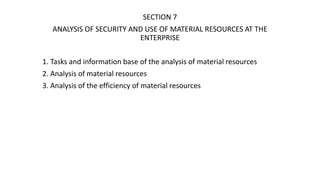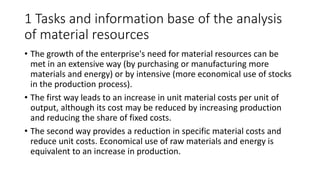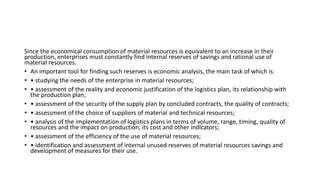Successfully reported this slideshow.×

# lecture 7.pptx

https://econ.biem.sumdu.edu.ua/

https://econ.biem.sumdu.edu.ua/

### lecture 7.pptx

1. 1. SECTION 7 ANALYSIS OF SECURITY AND USE OF MATERIAL RESOURCES AT THE ENTERPRISE 1. Tasks and information base of the analysis of material resources 2. Analysis of material resources 3. Analysis of the efficiency of material resources
2. 2. 1 Tasks and information base of the analysis of material resources • The growth of the enterprise's need for material resources can be met in an extensive way (by purchasing or manufacturing more materials and energy) or by intensive (more economical use of stocks in the production process). • The first way leads to an increase in unit material costs per unit of output, although its cost may be reduced by increasing production and reducing the share of fixed costs. • The second way provides a reduction in specific material costs and reduce unit costs. Economical use of raw materials and energy is equivalent to an increase in production.
3. 3. Since the economical consumption of material resources is equivalent to an increase in their production, enterprises must constantly find internal reserves of savings and rational use of material resources. • An important tool for finding such reserves is economic analysis, the main task of which is: • • studying the needs of the enterprise in material resources; • • assessment of the reality and economic justification of the logistics plan, its relationship with the production plan; • • assessment of the security of the supply plan by concluded contracts, the quality of contracts; • • assessment of the choice of suppliers of material and technical resources; • • analysis of the implementation of logistics plans in terms of volume, range, timing, quality of resources and the impact on production, its cost and other indicators; • • assessment of the efficiency of the use of material resources; • • identification and assessment of internal unused reserves of material resources savings and development of measures for their use.
4. 4. 2 Analysis of material resources • The cost of materials for production is calculated by the formula • Мв.в = 𝑖=1 𝑛 𝑁𝑖 ⋅ 𝑀ні ± 𝑀н.в • n – is the number of names of manufactured products; • Ni – the volume of output of the i-th name in the natural dimension; • Мні – the rate of material consumption per unit of the i-th product; • Мн.в. – material costs to change the balance of work in progress.
5. 5. • The company's need for material resources is determined by the formula • Мзаг = 𝑖=1 𝑛 М ⋅ Піj + Зі • Мзаг – the general need for material resources; • Піj – the need for the i-th type of materials for the production of the j-th type of product (based on the production program and the growth of work in progress); • Зі – necessary for the normal functioning of the enterprise stocks of the i-th type of material resources at the end of the period.
6. 6. • The supply of the enterprise with stocks in days is calculated as the ratio of the balance of this type of material resources to the average daily demand for this material: • МРі з – stock of the i-th type of material resources in physical units; • Ді п – one-day need for the i-th type of material resources in the same units. n і з і з і Д МР Д 
7. 7. • The degree of security of the need for material resources by contracts for their supply is assessed using the following indicators: • security ratio according to the plan Кзаб.пл • Кзаб.пл = Сума за укладеними договорами Планова необхідність • security ratio in fact Кзаб.ф • Кзаб.пл = Вартість факт. поставлених матер.ресурсів Планова необхідність
8. 8. • At the final stage of the analysis of supply of the enterprise with raw materials and materials the quantitative (cost) estimation of losses of production as a result is given: • - lack of resources; • - poor quality of materials; • - changes in prices for materials; • - downtime due to violation of the delivery schedule.
9. 9. • Violations in logistics affect the change in output, which can be determined by the formula • 𝛥ВП = П⋅Дв Др⋅Н • ВП – output; • П – the need for material for the program; • Дв – the number of days of absence of material; • Др – the number of working days in the reporting period; • Н – the rate of material consumption per product
10. 10. 3 Analysis of the efficiency of material resources • Analysis of the use of material resources should: • - to determine the actual situation regarding the cost of material resources at the enterprise; • - to reveal the reasons and conditions as a result of which a certain level in the expenditure of these resources was reached; • - to outline ways of further work on correction of shortcomings and economical use of materials, raw materials, fuel, energy.
11. 11. • The general indicators of the spent materials include: • • material efficiency; • • material consumption; • • the ratio of the growth rate of production and material costs; • • the share of material costs in the cost of production.
12. 12. • Material efficiency is determined by the ratio of product cost to the amount of material costs. This indicator characterizes the return of materials, namely - the output of each hryvnia of consumed material resources (raw materials, fuel, electricity): • Мвд = ВП Мв • ВП – commodity (gross) products; • Мв – material costs.
13. 13. • Material consumption is determined by the ratio of the amount of material costs to the cost of production and shows how much material costs per hryvnia of output: • Мм = Мв ВП • The ratio of the growth rate of production and material costs is determined by the ratio of the index of gross or marketable products to the index of material costs. It characterizes in relative terms the dynamics of material recovery and at the same time reveals the factors of its growth
14. 14. • The coefficient of material costs (the share of material costs in the cost of production) is the ratio of the actual amount of material costs to the planned, calculated on the actual volume of output. • It shows how economically materials are used in the production process, whether there is an overuse of them in comparison with the established norms.